# Combination

(diff) ← Older revision | Latest revision (diff) | Newer revision → (diff)

ofelements fromA subset of cardinalityof some given finite set of cardinality. The number of combinations ofelements fromis written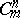orand is equal to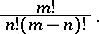The generating function for the sequence,,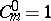, has the form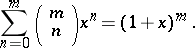Combinations can also be considered as non-ordered samples of sizefrom a general aggregate ofelements. In combinatorial analysis, a combination is an equivalence class of arrangements ofelements from(cf. Arrangement), where two arrangements of sizefrom a given-element set are called equivalent if they consist of the same elements taken the same number of times. In the case of arrangements without repetitions, every equivalence class is determined by the set of elements of an arbitrary arrangement from this class, and can thus be considered as a combination. In the case of arrangements with repetitions, one arrives at a generalization of the concept of a combination, and then an equivalence class is called a combination with repetitions. The number of combinations with repetitions offromis equal to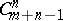, and the generating function for these numbers has the form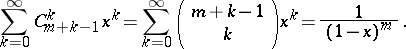How to Cite This Entry:
Combination. Encyclopedia of Mathematics. URL: http://encyclopediaofmath.org/index.php?title=Combination&oldid=33252
This article was adapted from an original article by V.M. Mikheev (originator), which appeared in Encyclopedia of Mathematics - ISBN 1402006098. See original article